10 Rates

# How to describe measurements properly

In all branches of science and technology people need to choose their vocabulary with care. Each term must have the same meaning for all of its users, and also express a well-defined concept which is not in conflict with everyday language. Every measurement is influenced by errors which are not completely known and this leads to measurement uncertainty. It is important to take account of this uncertainty when expressing the significance of a measurement. We must therefore precisely describe the impreciseness of the measurement itself.

The following article provides a set of important terms to be well understood and properly used by everyone involved in measurements.

## Calibration

A calibration is the comparison of achieved measurement results with a standard reference value. A calibration is performed to validate the quality of measurements and adjustments. The standard reference value is given by the certificate of density standard liquids, for instance.

Recommendation: 1 to 2 calibrations should be performed per year with certified standards.

## Adjustment

An adjustment is the modification of instrument constants to enable correct measurements and eliminate systematic measurement errors. An adjustment is carried out after a calibration, unless the stated deviation is within the tolerance range.

For the adjustment the density meter uses the density values of the standards and the measured oscillation periods to calculate the instrument constants. Usually two standards are required for an adjustment, like dry air and pure (e.g. bi-distilled), freshly degassed water.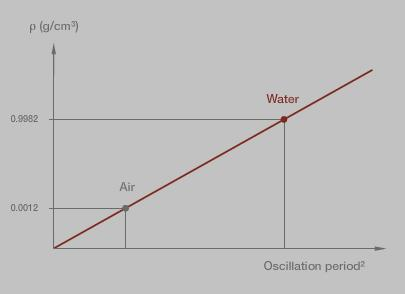Figure 1: Simplified adjustment graph of a density meter

## Measurement accuracy

The measurement accuracy expresses qualitatively how close the measurement result comes to the true value of a measurand.

In contrast, the quantitative measure of accuracy is uncertainty of measurement.

## Measurement precision

Measurement precision expresses qualitatively how close the measurement results come to each other under given measurement conditions.

Precision can be stated under repeatability or reproducibility conditions. Sometimes "measurement precision" is erroneously used to mean measurement accuracy.

Accuracy and precision states (from left to right) (Figure 2):

• Not accurate, not precise
• Accurate, not precise
• Not accurate, precise
• Accurate, precise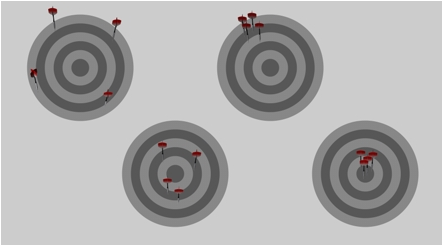Figure 2: Accuracy and precision states

## Uncertainty of measurement

The uncertainty of measurement specifies an interval within which the true value of the measurand is expected.

Uncertainty of measurement includes instrumental measurement uncertainty (arising from the measuring instrument), uncertainty of the calibration standards, and uncertainty due to the measurement process (sample preparation, sample filling,…).

Uncertainty of measurement generally comprises many components:

• Some of these components may be evaluated from the statistical distribution of the results or series of measurements and can be characterized by standard deviations (Type A evaluation according to ISO/IEC Guide 98-3:2008).
• The other components, which can also be characterized by standard deviations, are evaluated from assumed probability distributions based on experience or other information (Type B evalulation according to ISO/IEC Guide 98-3:2008).

## Instrumental measurement uncertainty

The instrumental measurement uncertainty is the component of measurement uncertainty arising from a measuring instrument or measuring system in use.

Instrumental measurement uncertainty is obtained through calibration of a measuring instrument or measuring system.

## Measurement repeatability

The measurement repeatability is the closeness of the agreement between the results of successive measurements of the same measurand carried out under the same conditions of measurement. Such ideal conditions lead to a minimum dispersion of measurement results.

The repeatability conditions are:

• The same measurement procedure
• The same operator
• The same measuring instrument, used under the same conditions
• The same location
• Repetition over a short period of time

Repeatability may be expressed with the repeatability standard deviation. This standard deviation is calculated from measurements carried out under repeatability conditions.

## Measurement reproducibility

Measurement reproducibility is the closeness of the agreement between the results of measurements of the same measurand carried out under changed conditions of measurement.

Such conditions lead to a maximum dispersion of measurement results.

These reproducibility conditions may include:

• Measuring principle
• Measuring method
• Operator
• Measuring instrument
• Reference standard
• Location
• Conditions of use
• Time

The changed measurement conditions have to be stated. Reproducibility may be expressed with the reproducibility standard deviation. This standard deviation is calculated from measurements carried out under defined reproducibility conditions.

## Measurement error

The measurement error is a measured quantity value minus a reference quantity value. Random measurement errors and systematic measurement errors can be distinguished.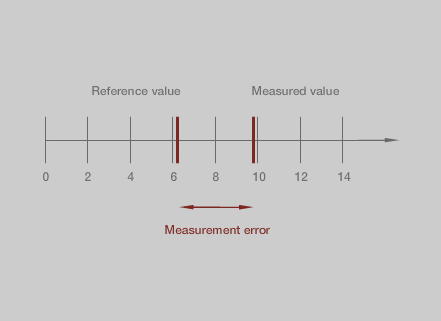Figure 3: Measurement error

## Random measurement error

The random measurement error is the component of a measurement error that varies in an unpredictable manner in replicated measurements.

Many measurements have to be carried out to eliminate random measurement errors. The mean value of these measurements tends towards the true value.

## Systematic measurement error

The systematic measurement error is the mean value that would result from an infinite number of measurements of the same measurand carried out under repeatability conditions, minus the true value of the measurand.

Systematic measurement errors, and their causes, are either known or unknown. A correction can be applied to compensate for a known systematic measurement error.

Measurement errors (from left to right) (Figure 4):

• Random measurement error
• Systematic measurement error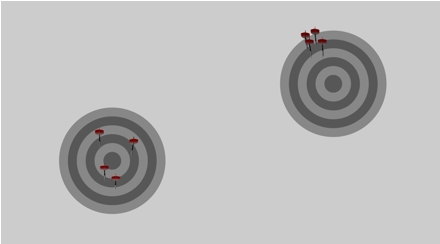Figure 4: Measurement errors

## Measurement bias

Measurement bias is the estimate of a systematic measurement error.

## Resolution

Resolution is the ability to resolve differences, i.e. to draw a distinction between two things. High resolution means being able to resolve small differences. In a digital system, resolution means the smallest increment or step that can be taken or seen. In an analog system, it means the smallest step or difference that can be reliably observed.

The most common mistake is the assumption that instruments with high resolution give more accurate results. High resolution does not necessarily mean high accuracy.

The accuracy of a system can never exceed its resolution!

Allegory for fine resolution:
With a fine marker it is possible to draw small dots.

Allegory for coarse resolution:
With a thick marker it is not possible to make fine drawings.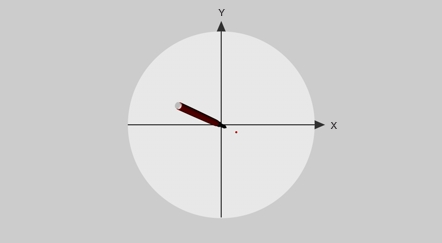Figure 5: Allegory for fine resolution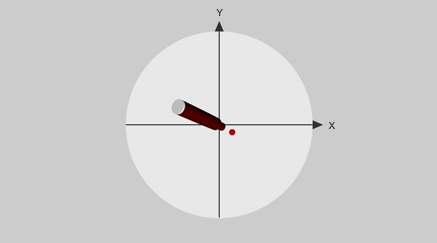Figure 6: Allegory for coarse resolution

## Arithmetic mean value

The arithmetic mean value x0 is the sum of the measurement values divided by the number of measurements n:

$$x _0 = {1 \over n} \sum_{i=1}^n x _i = {{x_1 + x_2 + ... + x_n} \over n}$$

Equation 1: arithmetic mean value
x0 … mean value
xi … measurement value of the ith measurement
n … number of measurements

The mean value does not give any information about the scattering of measurement results.

## Experimental standard deviation (s.d.)

For a series of n measurements of the same measurand, the experimental standard deviation s characterizes the dispersion of the results.

It is given by the formula:

$$s = \sqrt{{1 \over n-1} \sum _{i=1}^n (x _i - x_0)^2}$$

Equation 2: experimental standard deviation
s … empirical standard deviation
n … number of measurements
xi … measurement value of the ith measurement
x0 … arithmetic mean value

The mean value is often quoted along with the standard deviation. The mean value describes the central location of the data, the standard deviation describes the scattering.